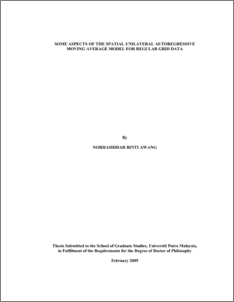# Some Aspects of the Spatial Unilateral Autoregressive Moving Average Model for Regular Grid Data

## Citation

Awang, Norhashidah (2005) Some Aspects of the Spatial Unilateral Autoregressive Moving Average Model for Regular Grid Data. PhD thesis, Universiti Putra Malaysia.

## Abstract

Spatial statistics has received much attention in the last three decades and has covered various disciplines. It involves methods which take into account the locational information for exploring and modelling the data. Many models have been considered for spatial processes and these include the Simultaneous Autoregressive model, the Conditional Autoregressive model and the Moving Average model. However, most researchers focused only on first-order models. In this thesis, a second-order spatial unilateral Autoregressive Moving Average (ARMA) model, denoted as ARMA(2,1;2,1) model, is introduced and some properties of this model are studied. This model is a special case of the spatial unilateral models which is believed to be useful in describing and modelling spatial correlations in the data. It is also important in the field of digital filtering and systems theory and for data whenever there is a natural ordering to the sites. Some explicit stationarity conditions for this model are established and some numerical computer simulations are conducted to verify the results. The general iv explicit correlation structure for this model over the fourth quadrant is obtained which is then specialised to AR(2,1), MA(2,1) and the second-order separable models. The results from simulation studies show that the theoretical correlations are in good agreement with the empirical correlations. A procedure using the maximum likelihood (ML) method is provided to estimate the parameters of the AR(2,1) model. This procedure is then extended to the case of spatial AR model of any order. For the AR(2,1) model, in terms of the absolute bias and the RMSE value, the results from simulation studies show that this estimator outperforms the other estimators, namely the Yule-Walker estimator, the ‘unbiased’ Yule-Walker estimator and the conditional Least Squares estimator. The ML procedure is then demonstrated by fitting the AR(1,1) and AR(2,1) models to two sets of data. Since the AR(2,1) model has the second-order terms which are only in one direction, two types of data orientation are taken into consideration. The results show that there is a preferred orientation of these data sets and the AR(2,1) model gives better fit. Finally, some directions for further research are given. In this research, inter alia, the field of spatial modelling has been advanced by establishing the explicit stationarity conditions for the ARMA(2,1;2,1) model, by deriving the explicit correlation structure over the fourth lag quadrant for ARMA(2,1;2,1) model and its special cases and by providing a modified practical procedure to estimate the parameters of the spatial unilateral AR model.Preview
PDF
1000548935_t_FS_2005_8.pdfView Item